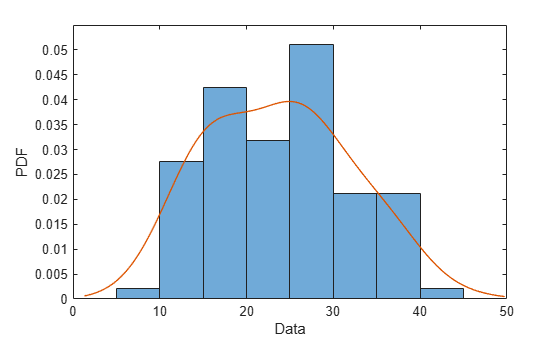# KernelDistribution

Kernel probability distribution object

## Description

A `KernelDistribution` object consists of parameters, a model description, and sample data for a nonparametric kernel-smoothing distribution.

The kernel distribution is a nonparametric estimation of the probability density function (pdf) of a random variable.

The kernel distribution uses the following options.

OptionDescriptionPossible Values
`Kernel`Kernel function type`normal`, `box`, `triangle`, `epanechnikov`
`Bandwidth`Kernel smoothing parameter`Bandwidth > 0`

## Creation

There are several ways to create a `KernelDistribution` probability distribution object.

## Properties

expand all

### Distribution Parameters

Kernel function type, specified as a valid kernel function type name.

Bandwidth of the kernel smoothing window, specified as a positive scalar value.

Data Types: `single` | `double`

### Distribution Characteristics

Logical flag for truncated distribution, specified as a logical value. If `IsTruncated` equals `0`, the distribution is not truncated. If `IsTruncated` equals `1`, the distribution is truncated.

Data Types: `logical`

Truncation interval for the probability distribution, specified as a vector of scalar values containing the lower and upper truncation boundaries.

Data Types: `single` | `double`

### Other Object Properties

Probability distribution name, specified as a character vector.

Data Types: `char`

Data used for distribution fitting, specified as a structure containing the following:

• `data`: Data vector used for distribution fitting.

• `cens`: Censoring vector, or empty if none.

• `freq`: Frequency vector, or empty if none.

Data Types: `struct`

## Object Functions

 `cdf` Cumulative distribution function `gather` Gather properties of Statistics and Machine Learning Toolbox object from GPU `icdf` Inverse cumulative distribution function `iqr` Interquartile range of probability distribution `mean` Mean of probability distribution `median` Median of probability distribution `negloglik` Negative loglikelihood of probability distribution `pdf` Probability density function `plot` Plot probability distribution object `random` Random numbers `std` Standard deviation of probability distribution `truncate` Truncate probability distribution object `var` Variance of probability distribution

## Examples

collapse all

Load the sample data. Visualize the patient weight data using a histogram.

```load hospital histogram(hospital.Weight)```The histogram shows that the data has two modes, one for female patients and one for male patients.

Create a probability distribution object by fitting a kernel distribution to the patient weight data.

`pd_kernel = fitdist(hospital.Weight,'Kernel')`
```pd_kernel = KernelDistribution Kernel = normal Bandwidth = 14.3792 Support = unbounded ```

For comparison, create another probability distribution object by fitting a normal distribution to the patient weight data.

`pd_normal = fitdist(hospital.Weight,'Normal')`
```pd_normal = NormalDistribution Normal distribution mu = 154 [148.728, 159.272] sigma = 26.5714 [23.3299, 30.8674] ```

Define the x values and compute the pdf of each distribution.

```x = 50:1:250; pdf_kernel = pdf(pd_kernel,x); pdf_normal = pdf(pd_normal,x);```

Plot the pdf of each distribution.

```plot(x,pdf_kernel,'Color','b','LineWidth',2); hold on; plot(x,pdf_normal,'Color','r','LineStyle',':','LineWidth',2); legend('Kernel Distribution','Normal Distribution','Location','SouthEast'); hold off;```Fitting a kernel distribution instead of a unimodal distribution such as the normal reveals the separate modes for the female and male patients.

## Version History

Introduced in R2013a